# QT Child Window from Main Window as Class

• Hello everyone,

I want to extend the QT Calculator Example so that I can perform numerical integrations.

I want to have a main Calculator window and a Child Integral window which opens when I click on the Integral Button.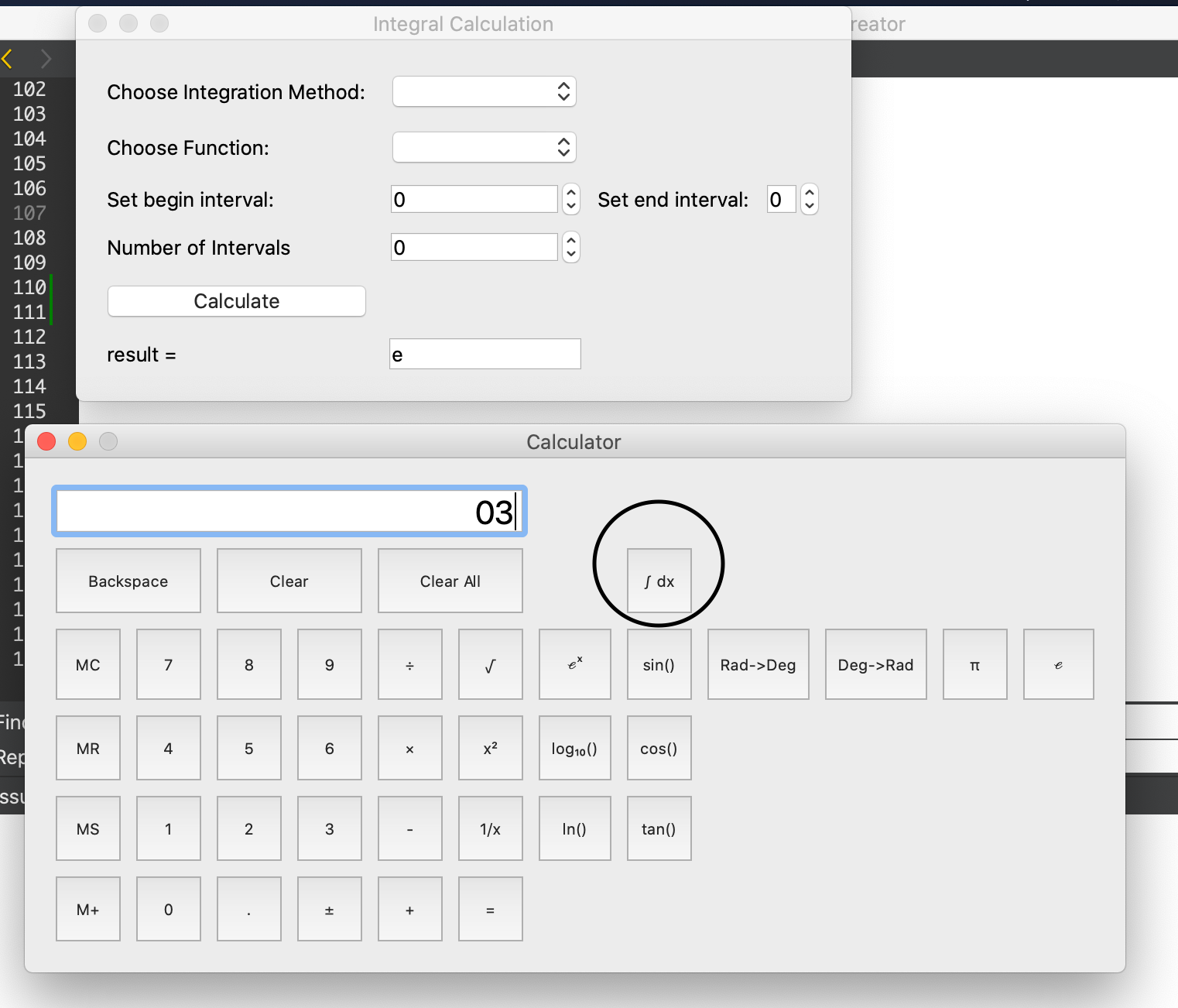Right Now the implementation of the Integral window is in the IntegralClicked Slot from the Main Calculator class.
BUt I want to create a child Integral Class and I don't know how.

this is the.h

class IntegralWindow: public QDialog{
Q_OBJECT
public:
explicit IntegralWindow(QWidget *parent=nullptr);
~IntegralWindow();
private:
QWidget *wdg;

};

class Calculator : public QWidget
{
Q_OBJECT

public:
Calculator(QWidget *parent = nullptr);

private slots:
void integralClicked();
//! 

//! 
private:
IntegralWindow *Int;

this is the .cpp

Calculator::Calculator(QWidget *parent)
: QWidget(parent), sumInMemory(0.0), sumSoFar(0.0)
, factorSoFar(0.0), waitingForOperand(true)
{...}

void Calculator::integralClicked(){

Int = new IntegralWindow(this);
Int->show();

}

Calculator.cpp

...
void Calculator::onIntegralClicked(){
IntegralWindow= new I
}

I get these symbol architecture undefined errors
Undefined symbols for architecture x86_64:
"IntegralWindow::IntegralWindow(QWidget*)", referenced from:
Calculator::integralClicked() in calculator.o
"IntegralWindow::~IntegralWindow()", referenced from:
vtable for IntegralWindow in moc_calculator.o
"IntegralWindow::~IntegralWindow()", referenced from:
vtable for IntegralWindow in moc_calculator.o
"non-virtual thunk to IntegralWindow::~IntegralWindow()", referenced from:
vtable for IntegralWindow in moc_calculator.o
"non-virtual thunk to IntegralWindow::~IntegralWindow()", referenced from:
vtable for IntegralWindow in moc_calculator.o
clang: error: linker command failed with exit code 1 (use -v to see invocation)
make: *** [calculator.app/Contents/MacOS/calculator] Error 1
08:32:09: The process "/usr/bin/make" exited with code 2.
Error while building/deploying project calculator (kit: Desktop Qt 5.14.2 clang 64bit)
When executing step "Make"

What exactly is wrong

Note that I have cut out the unneccessary parts
I also connected the integral clicked slot with the button (that;s not the problem)

• Hello, yes I could finally solve it.
I had got some problems with polymorphism

• IntegralWindow::IntegralWindow

You did not define it, did you?
Same for IntegralWindow::~IntegralWindow()

• Hello @jsulm
Right now I don't get these errors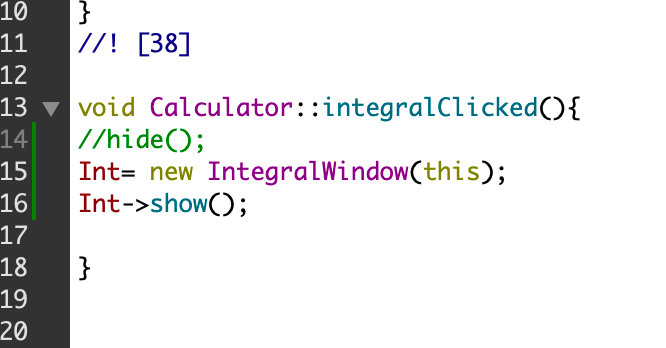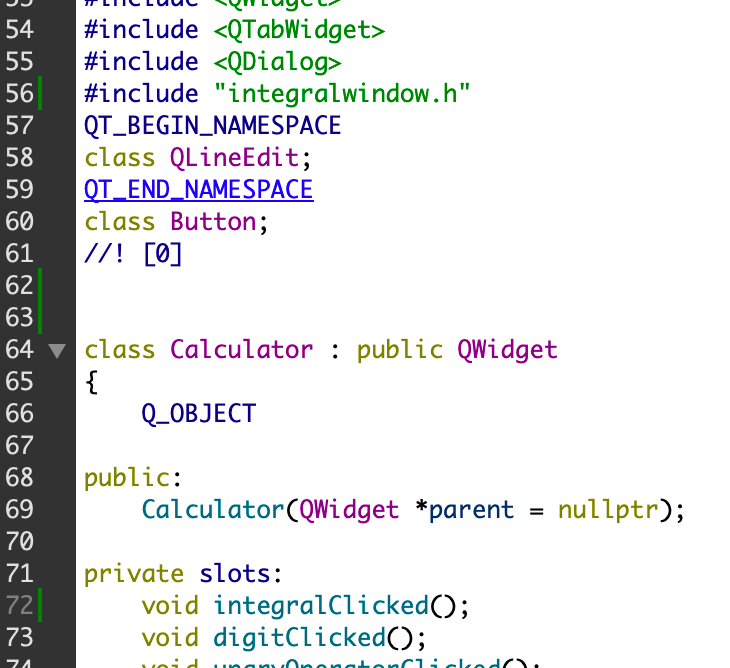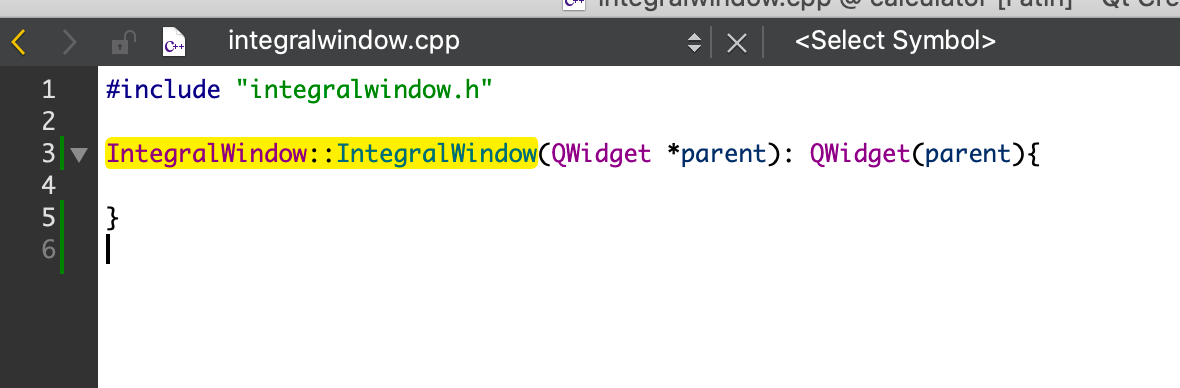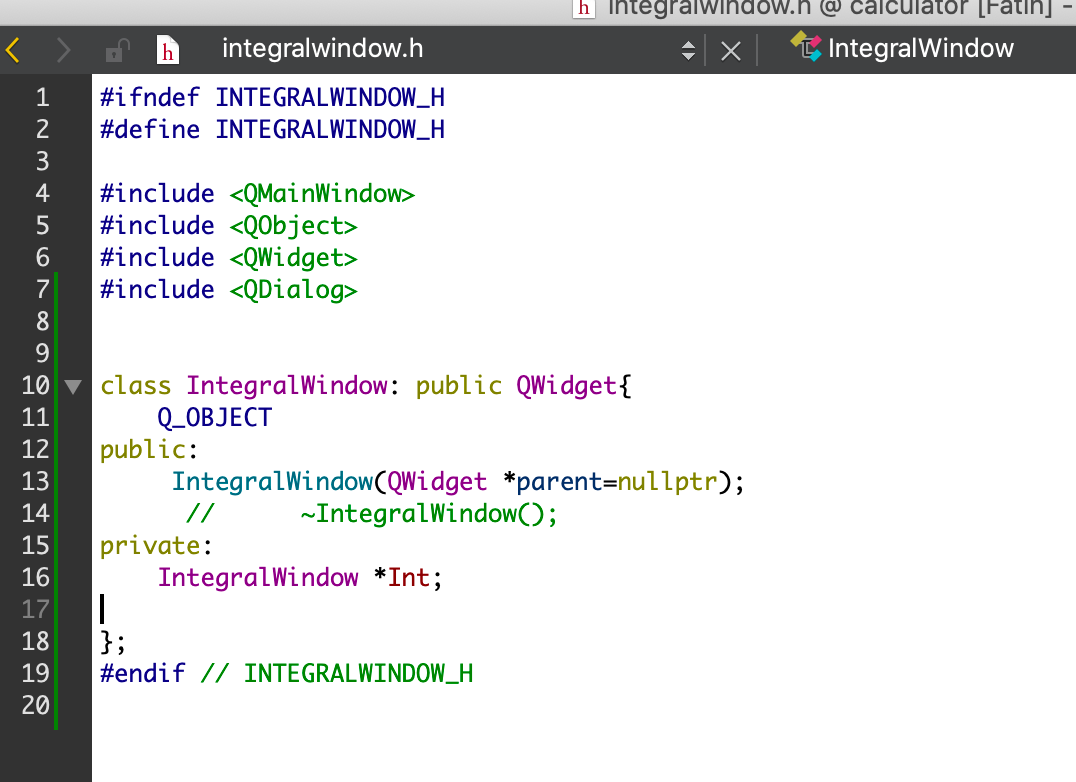BUt the window does not open

• @fatih1 Did you connect Calculator::integralClicked() to the signal from the button?

• Hello, yes I could finally solve it.
I had got some problems with polymorphism